• 傅里叶变换图像压缩的原理
千次阅读
2019-10-13 15:43:29

## 傅里叶变换实现图像压缩

题目
首先将图像分割为8x8的子图像，对每个子图像进行FFT.对每个子图像中的64个系数，排序后，舍去小的变换系数，只保留靠前的16个系数，实现4: I的图像压缩。

代码

t=imread('a6.jpg');
t=rgb2gray(t);%灰度化
[k,p]=size(t);
t=double(t)/255;%归一化 便于计算

%显示原图
imshow(t),title('原图','fontsize',16);

%利用blkproc 进行分块 并对每一块进行fft操作
t_fft=blkproc(t,[8 8],'fft2(x)');

%利用im2col进行优化操作 便于计算
t_block=im2col(t_fft,[8 8],'distinct');
[t_change,ix]=sort(t_block);%对每一块图像进行排序
[m,n]=size(t_block);

cr=0.75; %压缩比
nums=64-64*cr;

%对后48位系数清零
for i=1:n
t_block(ix(1:nums),i)=0;
end

t_rchange=col2im(t_block,[8 8],[k p],'distinct');
t_ifft=blkproc(t_rchange,[8 8],'ifft2(x)');%对每一块进行傅里叶反变换

figure,
imshow(t_ifft),title('8:1压缩后','fontsize',16);


效果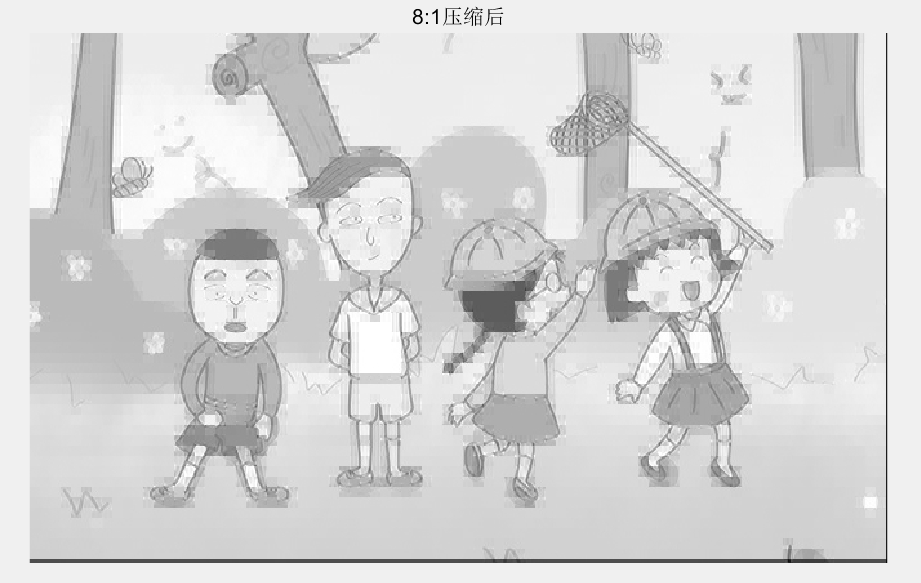MATLAB 数字图像处理 傅里叶变换图像压缩
更多相关内容
• 关于图像傅里叶变换，详情请见链接图像傅里叶变换博主大大讲的非常好。 cr = 0.025;% 表示压缩比为40倍 %读入并显示原始图像 I1 = imread('bar.bmp'); I1= rgb2gray(I1); I1 = double(I1)/255; %figure; %imshow...

关于图像的傅里叶变换，详情请见链接图像傅里叶变换博主大大讲的非常好。

cr = 0.025;% 表示压缩比为40倍
%读入并显示原始图像
I1= rgb2gray(I1);
I1 = double(I1)/255;
%figure;
%imshow(I1);title('ORI')
%对图像进行FFT
I1 = double(I1);
fftcoe = blkproc(I1, [8 8], 'fft2(x)');
coevar = im2col(fftcoe, [8 8], 'distinct');
coe = coevar;
[y, ind] = sort(coevar);
[m, n] = size(coevar);
snum = 64 - 64*cr;
%舍去不重要的系数
for i = 1: n
coe(ind(1: snum), i) = 0;
end
B2 = col2im(coe, [8 8], [512 512], 'distinct');
%对子图像块进行IFFT获得各个子图像的复原图像，并显示压缩图像
I2 = blkproc(B2, [8 8], 'ifft2(x)');
%figure;
%imshow(I2, [ ]);title('FFT');

实验在cr=10,20,40条件下进行：

原图：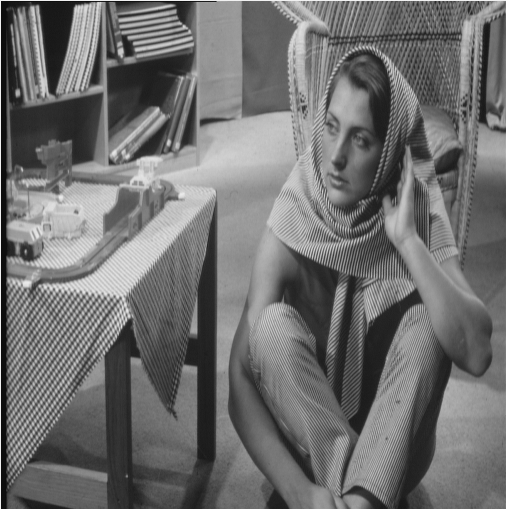1.cr=10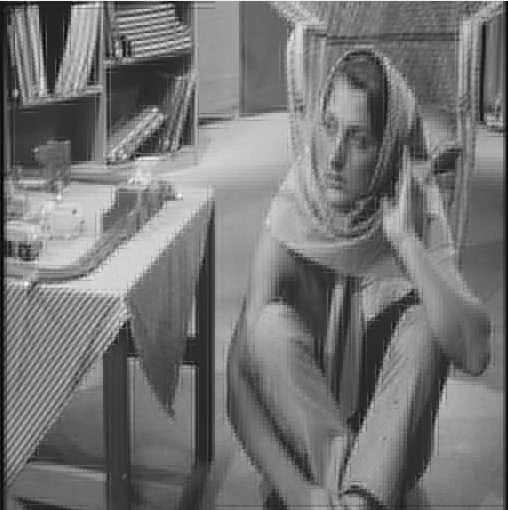2.cr=20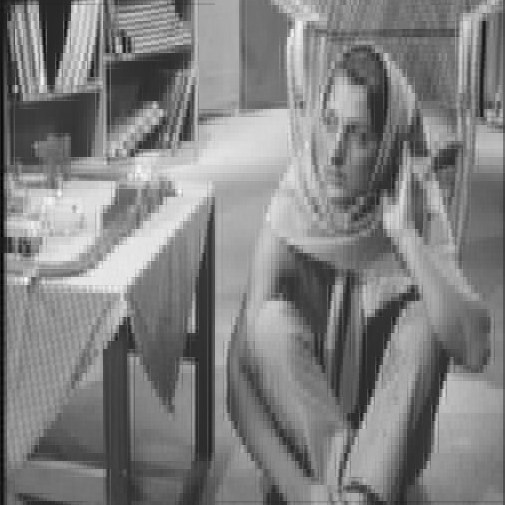3.cr=40展开全文图像压缩 傅里叶变换 计算机视觉
• 小波变换图像压缩方面的实现与应用一、实验图片的基本信息二、数据处理过程2.1小波函数的选择2.2图像压缩的基本思想三、不同小波函数压缩程度的对比四、MATLAB源码 一、实验图片的基本信息 小波变换作为一种新的...

# 一、实验图片的基本信息

小波变换作为一种新的数学工具，不仅继承了傅立叶变换的优点，同时又克服了它的许多缺点。由于小波变换是将图像分解成不同的频率子带。恰巧人眼对不同的频带的信息具有不同的响应，这一点人的视觉系统与小波很相似。数字图像压缩编码标准JPEG2000采用的就是小波变换技术。
为了验证小波变换技术在图像压缩方面的性能，现取一张手机拍摄的图片，用MATLAB将其转化为灰度图，如下所示（原始彩图和转变完的灰度图）。图片大小为41.1KB，像素为 375 × 500 375 \times 500 。（原图和源代码在我的上传资源“小波变换wavelet代码.rar”中）# 二、数据处理过程

## 2.1小波函数的选择

小波变换不同于傅里叶变换，根据小波母函数的不同，小波变换的结果也不尽相同。

从小波变换的原理出发，可以总结出一些选择标准：如支撑大小，对称性，正则性(平滑性)等。

支撑性：
大部分应用选择支撑长度为5~9之间的小波，因为支撑长度太长会产生边界问题，支撑长度太短精度太低，不利于信号能量的集中。

对称性：
具有对称性的小波，在图像处理中可以很有效地避免相位畸变。

正则性(平滑性):
在量化或者舍入小波系数时，为了减小重构误差对人眼的影响，必须尽量增大小波的光滑性或者连续可微性。也就是说正则性好的小波，能在信号或图像的重构中获得较好的平滑效果，减小量化或舍入误差的视觉影响。

本实验选用最基础的Haar小波函数、sym和JPEG2000标准中的Daubechies小波函数进行对比分析。它们都具有正交性，紧支撑性，对称性的小波函数。Haar小波函数的支撑长度为1，滤波器长度为2，消失矩阶数为1。Daubechies小波函数的支撑长度为2N-1，滤波器长度为2N，消失矩阶数为N-1。

## 2.2图像压缩的基本思想

小波变换用于图像压缩的基本思想就是根据二维小波分解算法，将一幅图像做小波分解，可得到一系列不同分辨率的图像。表现一幅图像最主要的部分是低频部分，而水平、垂直和对角线部分表征了原图像的边缘信息，具有明显的方向特性。低频部分可以称为亮度图像，水平、垂直和对角线部分可以称为细节图像。如果去掉图像的高频部分，保留低频部分，再根据人类的视觉生理和心理特点分别作不同策略的量化和编码处理，则可以达到图像压缩的目的。
在MATLAB中，**用wavedec2函数，对小波进行分解；用appcoef2函数，获得图像的近似信息（低频部分）；用detcoef2函数，获得图像在水平、垂直和对角方向的细节信息（高频部分）。**各方向信息如下图所示。利用wcodemat函数，对尺度1下的低频系数第一次压缩并对第一层信息进行量化编码。对尺度2下的低频系数做同样的操作，对图像进行第二次压缩。压缩后的图像如下图所示。# 三、不同小波函数压缩程度的对比

Haar和Daubechies小波函数压缩图像结果如下表所示。用sym5小波基得到压缩图像效果和Daubechies一样，就不再列出。

表1 两种不同的小波函数压缩图像结果从以上数据可以看出，两个方法的第一次压缩是提取原始图像中小波分解第一层的低频信息，此时压缩效果较好，压缩比较小约为1/4。第二次压缩是提取第一层分解低频部分的低频部分，即小波分解第二层的低频部分，其压缩比比较大，约为1/14。压缩效果在视觉上也基本过得去，它不需要经过其他处理即可获得较好的压缩效果。由表格可以看出，Haar小波变换的压缩性能稍微好于Daubechies（N=5）。当Daubechies小波函数中的N=1时为Haar小波。Haar小波在时域上是不连续的，所以作为基本小波性能不是特别好。Daubechies小波的特点是随着阶次（序列N）的增大消失矩阶数越大，其中消失矩越高光滑性就越好，频域的局部化能力就越强，频带的划分效果越好，所以在实际生活中的很多情景下Daubechies小波函数适用性更强。

# 四、MATLAB源码

% 数据压缩 ——图像压缩
% 2020.12.31 hx
close all;  clc; clear;
data=rgb2gray(data); whos('data');
figure; imshow(data);
title('原始图像');

% 小波分解
[c,s]=wavedec2(data,2,'sym3');
cA1=appcoef2(c,s,'sym3',1);%尺度1的低频系数
cA2=appcoef2(c,s,'sym3',2);%尺度2的低频系数
[cH1,cV1,cD1]=detcoef2('all',c,s,1);%尺度1的所有方向的高频系数H水平，V垂直，D对角
[cH2,cV2,cD2]=detcoef2('all',c,s,2);%尺度2的所有方向的高频系数
% wrcoef2  Reconstruct single branch from 2-D wavelet coefficients.
figure;
subplot(3,3,1); imshow(uint8(cA1));title('尺度1的低频系数图像'); %uint8的范围是0-255,转换
subplot(3,3,2); imshow(uint8(cA2));title('尺度2的低频系数图像');
subplot(3,3,4); imshow(uint8(cH1));title('尺度1水平方向高频系数图');
subplot(3,3,5); imshow(uint8(cV1));title('尺度1垂直方向');
subplot(3,3,6); imshow(uint8(cD1));title('尺度1斜线方向');
subplot(3,3,7); imshow(uint8(cH2));title('尺度2水平方向高频系数图');
subplot(3,3,8); imshow(uint8(cV2));title('尺度2垂直方向');
subplot(3,3,9); imshow(uint8(cD2));title('尺度2斜线方向');

%进行图像压缩
%第一层
cA1=wcodemat(cA1,192,'mat',0); %对矩阵进行量化编码
figure; subplot(121);
imshow(cA1,[]);  %将指定范围的灰度值变换到0-255区间显示
title('第一次压缩图像');
%第二层
cA2=wcodemat(cA2,192,'mat',0);
subplot(122); imshow(cA2,[]);
title('第二次压缩图像');
cA1=uint8(cA1);
cA2=uint8(cA2);
disp('第一次压缩后图像的大小：'); whos('cA1');
disp('第二次压缩后图像的大小：'); whos('cA2');


展开全文图像处理 matlab
• 实验目的 理解图像压缩的主要原则和目的，理解有损和无损压缩的概念;...求 RGB 彩色空间各个通道,彩色空间转换（RGB-HSI）,信号的傅里叶变换,图像的傅里叶变换,基于频域滤波的图像压缩,JPEG压缩算法中的DCT部分.

《多媒体技术与应用》实验报告

实验名称

实验二 数字图像压缩

实验时间

2022/4/11

姓名

班级

计非201

学号

成绩

• 实验目的
1. 理解图像压缩的主要原则和目的，理解有损和无损压缩的概念;
2. 了解几种常用的图像压缩编码方法;
3. 利用 Python 进行图像压缩算法验证。

二．实验原理

用 DCT 压缩图像的过程为： (1)首先将输入图像分解为 8×8 或 16×16 的块，然后对每个子块进行二维 DCT 变换。 (2)将变换后得到的量化的 DCT 系数进行编码和传送，形成压缩后的图像格式。 用 DCT 解压的过程为： (1)对每个 8×8 或 16×16 块进行二维 DCT 反变换。 (2)将反变换的矩阵的块合成一个单一的图像。

余弦变换具有把高度相关数据能量集中的趋势，DCT 变换后矩阵的能量集中在矩阵的 左上角，右下的大多数的 DCT 系数值非常接近于 0。对于通常的图像来说，舍弃这些接近 于 0 的 DCT 的系数值，并不会对重构图像的画面质量带来显著的下降。所以，利用 DCT 变换进行图像压缩可以节约大量的存储空间。压缩应该在最合理地近似原图像的情况下使用 最少的系数。使用系数的多少也决定了压缩比的大小。 在压缩过程的第 2 步中，可以合理地舍弃一些系数，从而得到压缩的目的。在压缩过程的第 2 步，还可以采用 RLE 和 Huffman来进一步压缩.

三．实验内容与关键步骤

求 RGB 彩色空间各个通道：

彩色空间转换（RGB-HSI）：

信号的傅里叶变换

图像的傅里叶变换

基于频域滤波的图像压缩

JPEG 压缩算法中的 DCT 部分

四．代码

1. 求 RGB 彩色空间各个通道

from skimage import data
from matplotlib import pyplot as plt
import numpy as np

image = data.logo()  # 载入测试图像
fig = plt.figure()
plt.rcParams['font.sans-serif'] = ['SimHei']
plt.rcParams['axes.unicode_minus'] = False
# 两行两列的第一个子图
plt.axis('off')  # 不显示坐标轴
plt.imshow(image)  # 显示RGB彩色图像
plt.title('RGB图像')
# 第二个子图
imageR = image[:, :, 0]
plt.axis('off')
plt.imshow(imageR, cmap='gray')  # 显示R通道图像
plt.title('R通道图像')
# 第三个子图
imageG = image[:, :, 1]
plt.axis('off')
plt.imshow(imageG, cmap='gray')  # 显示G通道图像
plt.title('G通道图像')
# 第四个子图
imageB = image[:, :, 2]
plt.axis('off')
plt.imshow(imageB, cmap='gray')  # 显示B通道图像
plt.title('B通道图像')
plt.show()
# plt.savefig('RGB通道图像.tif')   #也可以把结果保存为图像文件


2. 彩色空间转换（RGB-HSI）

import skimage
from matplotlib import pyplot as plt
import math
import numpy as np
import sys
from skimage import io

# 定义RGB转HSI
def rgb2hsi(r, g, b):
r = r / 255
g = g / 255
b = b / 255
h = 0
num = 0.5 * ((r - g) + (r - b))
den = ((r - g) * (r - g) + (r - b) * (g - b)) ** 0.5

if b <= g:
if den == 0:
den = sys.float_info.min
h = math.acos(num / den)
elif b > g:
if den == 0:
den = sys.float_info.min
h = (2 * math.pi) - math.acos(num / den)

s = 1 - (3 * min(r, g, b) / (r + g + b))
i = (r + g + b) / 3

return int(h), int(s * 100), int(i * 255)

# 注意skimage中的图片读取方式
hsi_image = np.zeros(image.shape, dtype='uint8')
for ii in range(image.shape):
for jj in range(image.shape):
r, g, b = image[ii, jj, :]
h, s, i = rgb2hsi(r, g, b)
hsi_image[ii, jj, :] = (h, s, i)

plt.rcParams['font.sans-serif'] = ['SimHei']
plt.rcParams['axes.unicode_minus'] = False
plt.subplot(2, 3, 1)
plt.axis('off')
plt.imshow(image)
plt.title('RGB原图像')
plt.subplot(2, 3, 2)
plt.axis('off')
plt.imshow(image[:, :, 0], cmap='gray')
plt.title('R分量')
plt.subplot(2, 3, 3)
plt.axis('off')
plt.imshow(hsi_image)
plt.title('HSI图像')
plt.subplot(2, 3, 4)
plt.axis('off')
plt.imshow(hsi_image[:, :, 0], cmap='gray')
plt.title('H分量')
plt.subplot(2, 3, 5)
plt.axis('off')
plt.imshow(hsi_image[:, :, 1], cmap='gray')
plt.title('S分量')
plt.subplot(2, 3, 6)
plt.axis('off')
plt.imshow(hsi_image[:, :, 2], cmap='gray')
plt.title('I分量')
plt.savefig('HSIimage2.tif')


3. 信号的傅里叶变换

import matplotlib.pyplot as plt
import numpy as np

"""
中文显示工具函数
"""

def set_ch():
from pylab import mpl
mpl.rcParams['font.sans-serif'] = ['FangSong']
mpl.rcParams['axes.unicode_minus'] = False

set_ch()

def show(ori_func, ft, sampling_period=5):
n = len(ori_func)
interval = sampling_period / n
# 绘制原始函数
plt.subplot(2, 1, 1)
plt.plot(np.arange(0, sampling_period, interval), ori_func, 'black')
plt.xlabel('时间'), plt.ylabel('振幅')
plt.title('原始信号')
# 绘制变换后的函数
plt.subplot(2, 1, 2)
frequency = np.arange(n / 2) / (n * interval)
nfft = abs(ft[range(int(n / 2))] / n)
plt.plot(frequency, nfft, 'red')
plt.xlabel('频率 (Hz)'), plt.ylabel('频率谱')
plt.title('傅里叶变换结果')
plt.subplots_adjust(left=None, bottom=None, right=None, top=None, wspace=None, hspace=0.9)  # 调整子图间距
plt.show()

# 生成频率为 1（角速度为 2 * pi）的正弦波
time = np.arange(0, 5, .005)
x = np.sin(5 * np.pi * 3 * time)
y = np.fft.fft(x)
show(x, y)


4. 图像的傅里叶变换

from skimage import data
import numpy as np
from matplotlib import pyplot as plt

"""
中文显示工具函数
"""

def set_ch():
from pylab import mpl
mpl.rcParams['font.sans-serif'] = ['FangSong']
mpl.rcParams['axes.unicode_minus'] = False

set_ch()

img = data.coins()
f = np.fft.fft2(img)  # 快速傅里叶变换算法得到频率分布
fshift = np.fft.fftshift(f)  # 默认结果中心点位置是在左上角，转移到中间位置

fimg = np.log(np.abs(fshift))  # fft 结果是复数，求绝对值结果才是振幅

# 展示结果
plt.subplot(121), plt.imshow(img, 'gray'), plt.title('原始图像')
plt.subplot(122), plt.imshow(fimg, 'gray'), plt.title('傅里叶频谱')
plt.show()

5. 基于频域滤波的图像压缩

# 导入相关库
from skimage import data, color
import numpy as np
import matplotlib.pyplot as plt

"""
中文显示工具函数
"""

def set_ch():
from pylab import mpl
mpl.rcParams['font.sans-serif'] = ['FangSong']
mpl.rcParams['axes.unicode_minus'] = False

set_ch()

D = 10
# 读入图片
new_img = data.astronaut()
new_img = color.rgb2gray(new_img)

# numpy中的傅里叶变化
f1 = np.fft.fft2(new_img)
f1_shift = np.fft.fftshift(f1)
# np.fft.fftshift()函数来实现平移,让直流分量在输出图像的重心

# 实现理想低通滤波器
rows, cols = new_img.shape
crow, ccol = int(rows / 2), int(cols / 2)  # 计算频谱中心
mask = np.zeros((rows, cols), np.uint8)  # 生成rows行cols的矩阵，数据格式为uint8
for i in range(rows):
for j in range(cols):
if np.sqrt(i * i + j * j) <= D:
# 将距离频谱中心小于D的部分低通信息 设置为1，属于低通滤波
mask[crow - D:crow + D, ccol - D:ccol + D] = 1
f1_shift = f1_shift * mask

# 傅里叶逆变换
f_ishift = np.fft.ifftshift(f1_shift)
img_back = np.fft.ifft2(f_ishift)
img_back = np.abs(img_back)
img_back = (img_back - np.amin(img_back)) / (np.amax(img_back) - np.amin(img_back))

# plt.figure(figsize=(15,8))
plt.figure()
plt.subplot(121), plt.imshow(new_img, cmap='gray'), plt.title('原始图像')
plt.subplot(122), plt.imshow(img_back, cmap='gray'), plt.title('滤波后图像')
plt.show()


6. JPEG 压缩算法中的 DCT 部分

# 基于离散余弦变换DCT的图像压缩
import cv2
import numpy as np
import matplotlib.pyplot as plt

img = cv2.imread('coffee.png', 0)  # 读取图片

img1 = img.astype('float')  # 将uint8转化为float类型

img_dct = cv2.dct(img1)  # 进行离散余弦变换

img_dct_log = np.log(abs(img_dct))  # 进行log处理

img_recor = cv2.idct(img_dct)  # 进行离散余弦反变换

# 图片压缩，只保留100*100的数据
recor_temp = img_dct[0:10, 0:10]
recor_temp2 = np.zeros(img.shape)
recor_temp2[0:10, 0:10] = recor_temp
# 压缩图片恢复
img_recor1 = cv2.idct(recor_temp2)
# 显示
plt.subplot(221)
plt.imshow(img)
plt.title('original')

plt.subplot(222)
plt.imshow(img_dct_log)
plt.title('dct transformed')

plt.subplot(223)
plt.imshow(img_recor)
plt.title('idct transformed')

plt.subplot(224)
plt.imshow(img_recor1)
plt.title('idct transformed2')
plt.subplots_adjust(left=None, bottom=None, right=None, top=None, wspace=None, hspace=0.9)  # 调整子图间距
plt.show()


五．实验结果与分析

 求 RGB 彩色空间各个通道 Coffee Retina Logo 结果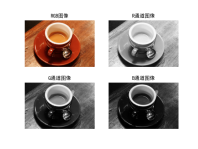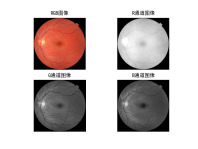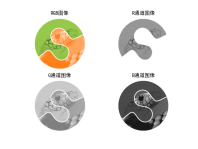彩色空间转换（RGB-HSI） chelsea Flower lenacolor 结果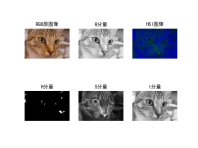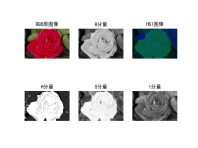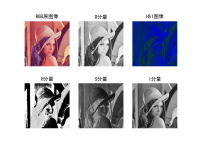信号的傅里叶变换 频率为 1（角速度为 2 * pi）的正弦波 频率为 1（角速度为 3 * pi）的正弦波 频率为 5（角速度为 3 * pi）的正弦波 结果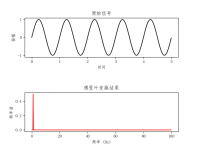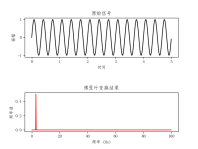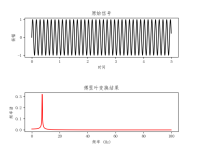图像的傅里叶变换 Camera Brick Coins 结果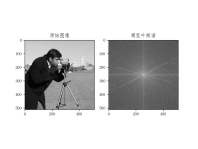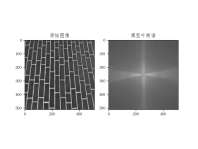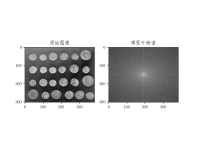基于频域滤波的图像压缩 Coffee chelsea astronaut 结果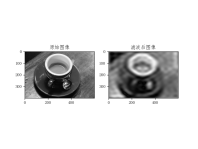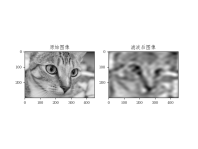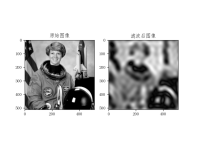JPEG 压缩算法中的 DCT 部分 保留100*100 保留10*10 结果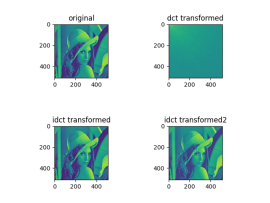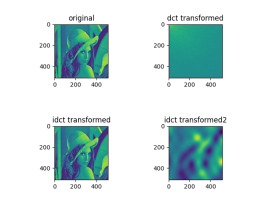最后一个实验如果提示cv2第三方库找不到,可以试试安装opencv-contrib-python这个,安装完就可以了

六．实验心得体会

通过本次实验,我明白了怎么进行彩色空间转化,如何对图像进行压缩以及如何进行图像和信号的傅里叶变换的操作.对图像压缩的原理有有了新的体会.

展开全文python
• 点击上方“小白学视觉”，选择加"星标"或“置顶”重磅干货，第一时间送达图 (a): (从左到右) (1) 原始图片 (2) 使用高斯低通滤波器 (3) 使用高斯高通滤波器. 本文中的原始图...
• 基于压缩感知和离散傅里叶变换的加密图像中可分离数据隐藏
•图像压缩
• 傅立叶变换是将图像从空间域转换到频率域，其逆变换是将图像从频率域转换到空间域。python 图像处理 计算机视觉
• ## 图像处理（5）--图像的傅里叶变换

万次阅读 多人点赞 2019-07-06 10:42:52
傅里叶变换及其反变换1.1 为什么要在频率域研究图像增强？1.2 傅里叶变换 1. 傅里叶变换及其反变换 1.1 为什么要在频率域研究图像增强？ 可以利用频率成分和图像外表之间的对应关系。一些在空间域表述困难的增强...图像处理 傅里叶变换
• 小波变换是在短时傅里叶变换的基础上发展起来的一种新型变换方法，他是一种时—...小波变换由于具有很好的时—频特性而且可以匹配人类视觉系统的特性，因而得到图像压缩编码领域的极大关注。小波分析在图像领域的应...
• 1、为什么要进行图像压缩       众所周知，当今人类社会具有三大支柱，即物质、能量、信息。当下已由物质过渡到信息，从农业现代化到工业现代化，再到当今的信息化时代。信息具有通用性、抽象性...
• 正交变换图像压缩 傅里叶变换 时域连续—>频域非周期 时域的非周期—>频域连续 ​ 图像的频率—>图像中灰度变化的剧烈程度的指标。 傅里叶频谱图上看到的亮点实际是图像上某一点与领域点差异的...数字图像处理
•  离散余弦变换归一化的二维傅里叶变换如下：从傅里叶变换到离散余弦变换，需要一些数学理论的支持。在给定区间内满足狄利赫里条件的连续实对称函数，可以展开成仅含有余弦项的傅里叶级数。以一个二维离散函数f(x,y)...图像处理 图像压缩
• 文章目录傅里叶变换概念定义帕瑟瓦尔定理 傅里叶变换概念 傅里叶变换（Fourier transform）是一种线性积分变换，用于信号在时域（或空域）和频域之间的变换。 将函数（通常是时间的函数或信号）分解为三角函数（正弦...图像处理 离散时间傅立叶变换
• 傅里叶变换的方法对彩色图像进行压缩，适合初学者
• 提出了一种新的基于信息光学的图像数字水印...理论分析和数值计算实验表明,该水印技术对有损压缩、剪切和滤波等多种图像处理操作均具有很高的稳健性,比传统傅里叶变换全息水印的稳健性有很大提高,具有很好的实用价值。
• 本文采用MATLAB软件编写程序,利用一维或二维的傅里叶变换函数,实现了对二维图像信息的傅里叶变换,通过程序对其频谱结果进行处理,利用傅里叶变换函数,实现了对二维图像信息的压缩,进而展示了傅里叶变换与反变换在...
• 二维离散傅里叶变换是将图像从空间域转至频域，在图像增强、图像去噪、图像边缘检测、图像特征提取、图像压缩等等应用中都起着极其重要的作用。理论基础是任意函数都可以表示成正弦函数的线性组合的形式。公式如下 ...图像处理 傅里叶变换 幅度谱 频域变换
• 沈阳理工大学数字图像处理报告PAGE 10数字图像傅里叶变换课程设计目的(1)了解图像变换的意义和手段(2)熟悉傅里叶变换的基本性质(3)热练掌握FFT的方法反应用(4)通过本实验掌握利用MATLAB编程实现数字图像傅里叶...
• 图像傅里叶变换的物理意义：图像的频率是表征图像中灰度变化剧烈程度的指标，是灰度在平面空间上的梯度。如：大面积的沙漠在图像中是一片灰度变化缓慢的区域，对应的频率值很低；而对于地表属性变换剧烈的边缘区域在...
• ## JPEG 图像压缩原理

千次阅读 2019-10-26 20:24:17
JPG格式的图片体积相对较小，是因为它采用了一系列的压缩算法，压缩图片弊端就是和原始的图片相比，它牺牲掉了一些画面细节，这些丢失的细节或许可被人的肉眼看出，或许以人的肉眼难以发现，对于这种通过牺牲画面的...JPEG压缩
• 展开全部冈萨雷斯版里面的解释非常形象：一个恰当的比喻是将傅里叶变换比作62616964757a686964616fe58685e5aeb931333361303635一个玻璃棱镜。棱镜是可以将光分解为不同颜色的物理仪器，每个成分的颜色由波长(或频率)...
• 一维傅里叶变换原理可以通俗的理解为：将一个复杂无规律的信号拆分成多个简单有规律的子信号来表示。 为了定量表示这个结果，以横轴为频率大小，纵轴为振幅（即信号的最高强度），此图称为信号的频谱。 频谱中的每...傅立叶变换 灰度值 图像高频分量
• 傅立叶变换图像处理中有非常非常的作用。因为不仅傅立叶分析涉及图像处理的很多方面，傅立叶的改进算法， 比如离散余弦变换，gabor与小波在图像处理中也有重要的分量。 印象中，傅立叶变换图像处理以下几个...
• 根据图像傅立叶变换域频谱系数特征,提出了一种基于DFT的数字图像压缩算法,并通过仿真实验对图像进行了不同压缩比的压缩。实验结果表明:衡量编码压缩质量的5个参数和评价压缩图像逼真度的参数均达到较好效果,且即使...
• 傅里叶变换是应用最广泛的一种频率变换，它能够将图像从空间域变换到频率域，而傅里叶变换能够将频率域信息变换到空间域内。在数学界的含义是任何周期函数都可以表示为不同频率的正弦函数和的形式。在图像处理过程...计算机视觉 opencv 人工智能 图像处理 python
• 频域处理图像变换频域与频域变换傅立叶的两个最主要的贡献傅立叶变换(一种正交变换)连续函数的傅立叶变换例子离散傅立叶变换一维离散傅立叶变换的计算举例1-D傅里叶变换矩阵格式2-D傅里叶变换矩阵表示例子离散傅立叶...图像处理
• 图像的正交变换在数字图像的处理与...1. 傅里叶变换实验原理对一幅图像进行 离散傅里叶变换 (DFT)，可以得到图像信号的傅里叶频谱。二维 DFT 的变换及逆变换公式如下：DFT 尽管解决了频域离散化的问题，但运算量太......